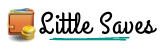# Compound Interest Calculator – Calculate interest in Rupees online

The compound interest calculator helps you calculate recurring interest you receive on a fixed investment in India over a period of time in Indian Rupees.Here is a simple compound interest calculator to find out the interest you receive in Rupees. This is tailored for Indians to calculate the returns on investments in India on a recurring and compounding basis.

How much is the principal you are investing?
Field is required!
Field is required!
What is the duration of the investment
Field is required!
Field is required!
How often is the Interest paid out
• - select a option -
• Monthly
• Yearly
• Quarterly
• Half Yearly
• Weekly
• Fortnightly (every 2 weeks)
Field is required!
Field is required!
Rate of interest of the investment (in %)
Invalid Rate of Interest
Invalid Rate of Interest
Rate of Interest you mentioned, is it Per Month (pm) or Per Annum (pa)
Field is required!
Field is required!
Maturity Amount
Maturity Amount0.00
Field is required!
Field is required!

## How to calculate Compound Interest Online

This calculator is pretty straight forward. Here are the elements used to calculate your interest and what values you need to enter in them to find out your interest.

### Principal

This is the amount of money in Indian Rupees(INR) you are investing on which you are expecting a return on

### Duration

This is how long you are making this investment or deposit for. Mention this as years. Eg: If you are investing for 6 months, mention 0.5 as the duration of investment

### Interest Payout Cycle

How often is the interest paid out. This can be weekly, forthnightly, monthly, quarterly, half-yearly or annually.

### Rate of Interest

What is the % rate of interest that will be paid on this investment.

### Frequency of Interest

The rate of interest you selected, is it monthly or annual interest. Eg: if 5%, is it 5% per month or 5% per year

What is the formula for calculating compound interest

A = P(1+r/n)nt
Here: A = the final amount you receive after maturity
P = principal or initial amount of money you invested
r = rate of interest you are getting on the investment
n = frequency of interest payout
t = duration of the investment

What is the difference between Simple interest and compound interest

Investments on Simple interest earn fixed rate of interest and the interest earned does not generate further interest. Eg: In compound interest, for Rs.10,000 loan at 1% per month, the first month, interest is Rs.100, second month interest will be Rs.101. Rs.100 for the initial investment of Rs.10,000 and another Rs.1 as 1% interest on Rs.100 interest earned from the previous month.
In Simple interest, for the same scenario, every month, you earn Rs.100 that accumulates till maturity

Which is better, Simple Interest or Compound Interest

Compound interest is better as it has a higher rate of return and will give you more money. Refer to example above.

What is the difference between recurring and compound interest

Both are the same, compound interests are recurring in nature till maturity.

## Wait!

#### Before you go, take a copy of our detailed Finance Planner worth \$39 for free#### Abhi

Abhi is a 29 yr old Indian, on FIRE to retire by 40. He has been investing and learning Finance for the past 12 years. After completing Mechanical engineering, he started working in a multi-national Bank and grew to become an AVP. Currently with an IT MNC as a VP. He lives in Bangalore with his wife and their 1 year old daughter. In his free time, Abhi loves to game on the Xbox, watch movies, read and blog.

Subscribe
Notify of